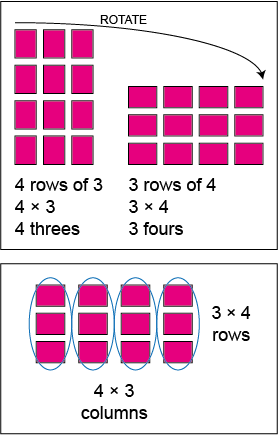Home > Mental computation > Big ideas > Generalising patterns and relationships > Commutative and associative properties

# Commutative and associative properties

Knowing the commutative property of addition and multiplication reduces the number of facts to be learned (e.g. 3 $$\times$$ 6 = 18, so 6 $$\times$$ 3 = 18).

The commutative property of addition and multiplication is often referred to as the 'turn around' property because the order of adding or multiplying the numbers does not affect the answer.

For example, to work out 4 + 7 = ? the student recognises that 7 + 4 = ? is easier to work out by using the 'build to ten' strategy. Similarly, when given 6 + 19 = ? adding on 6 to 19 is easier than adding on 19 to 6.

The commutative property for multiplication is not as intuitively obvious as that of addition, particularly using the set model.

The rectangular array model can enable students to see the connection and recognise why they have the same product.Array models illustrating commutative processes for multiplication.

The associative property of addition and multiplication can be used when adding or multiplying more than two numbers.

For example, to work out 13 + 18 + 2 it is easier to add 18 + 2 first than proceed from left to right.

To work out the product of 4 $$\times$$ 6 $$\times$$ 5 it is easier to calculate the 6 $$\times$$ 5 part first then multiply 30 by 4.